# Control Flow III Control Flow Optimizations EECS 483

• Slides: 30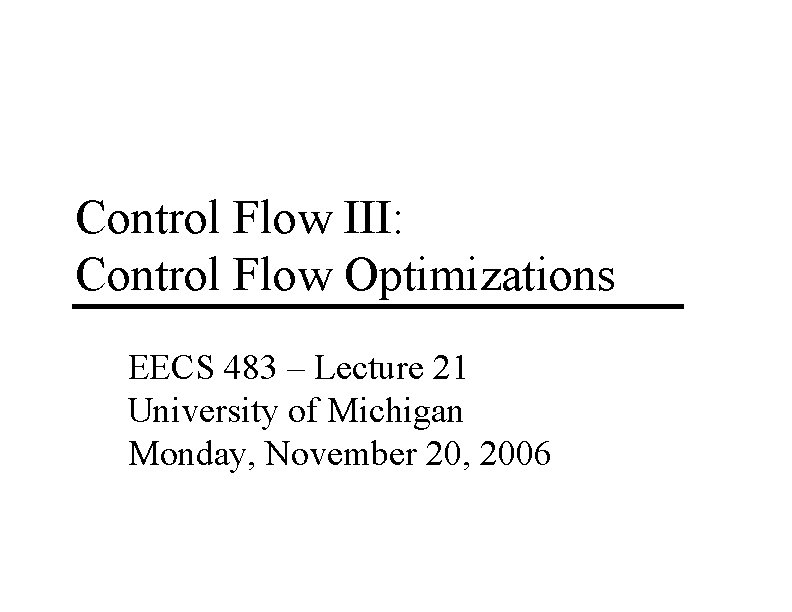Control Flow III: Control Flow Optimizations EECS 483 – Lecture 21 University of Michigan Monday, November 20, 2006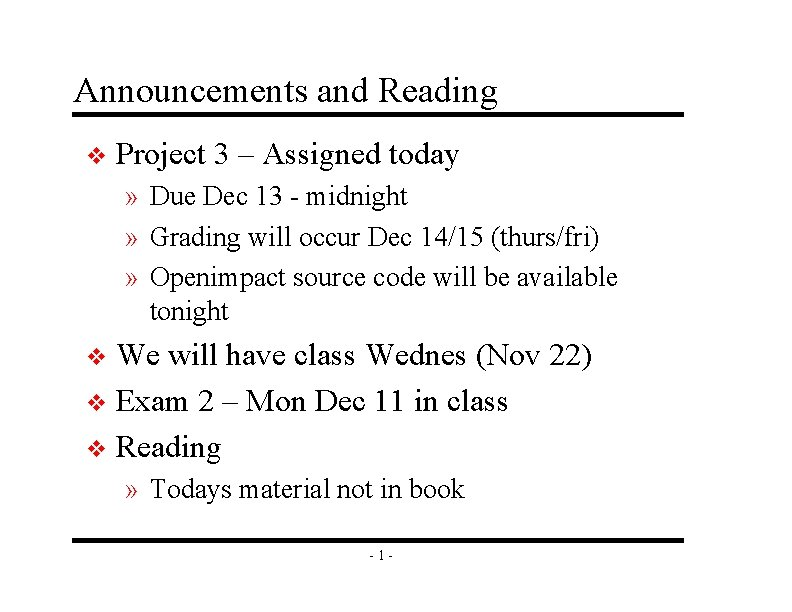Announcements and Reading v Project 3 – Assigned today » Due Dec 13 - midnight » Grading will occur Dec 14/15 (thurs/fri) » Openimpact source code will be available tonight We will have class Wednes (Nov 22) v Exam 2 – Mon Dec 11 in class v Reading v » Todays material not in book -1 -Project 3 Compiler System - Openimpact Lemulate C code to test functional correctness at any point Sample appln input and output C Frontend (Pcode) Backend (Lcode) Pto. L Parsing, syntax check Profiling Heuristic function inlining (disabled) Memory dependence analysis Control flow analysis Dataflow analysis Classical optimizations Profiling Scheduling Register allocation Cycle count estimates -2 - Lcode asmCompilation Steps v 1. EDG frontend – » Lexical/syntax/semantic analysis » Pcode is created v 2. Pcode flattening » remove complex control flow (ie &&, || operators) v 3. Pcode profiling » Note – compilation not halted if profile fails v 4. Pcode inlining (disabled by default) » Ask me if you want to enable it v v 5. Interprocedural pointer analysis 6. Lcode generation (Pto. L) » Generates *. lc files, textual assembly, this is the input to your optimizations -3 -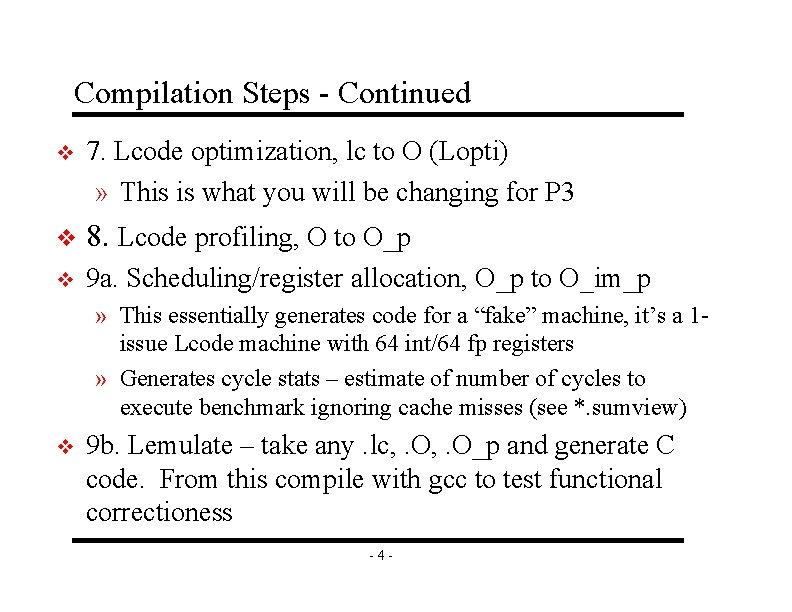Compilation Steps - Continued v 7. Lcode optimization, lc to O (Lopti) » This is what you will be changing for P 3 v 8. Lcode profiling, O to O_p v 9 a. Scheduling/register allocation, O_p to O_im_p » This essentially generates code for a “fake” machine, it’s a 1 issue Lcode machine with 64 int/64 fp registers » Generates cycle stats – estimate of number of cycles to execute benchmark ignoring cache misses (see *. sumview) v 9 b. Lemulate – take any. lc, . O_p and generate C code. From this compile with gcc to test functional correctioness -4 -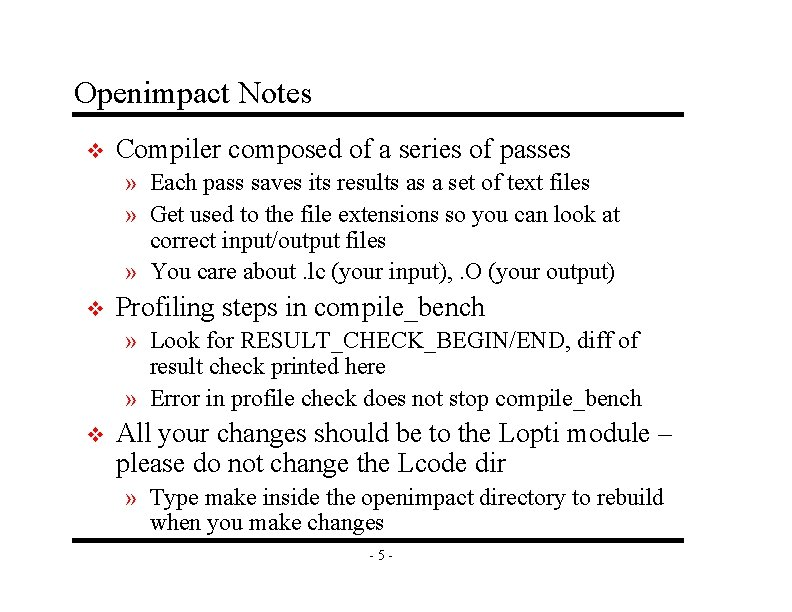Openimpact Notes v Compiler composed of a series of passes » Each pass saves its results as a set of text files » Get used to the file extensions so you can look at correct input/output files » You care about. lc (your input), . O (your output) v Profiling steps in compile_bench » Look for RESULT_CHECK_BEGIN/END, diff of result check printed here » Error in profile check does not stop compile_bench v All your changes should be to the Lopti module – please do not change the Lcode dir » Type make inside the openimpact directory to rebuild when you make changes -5 -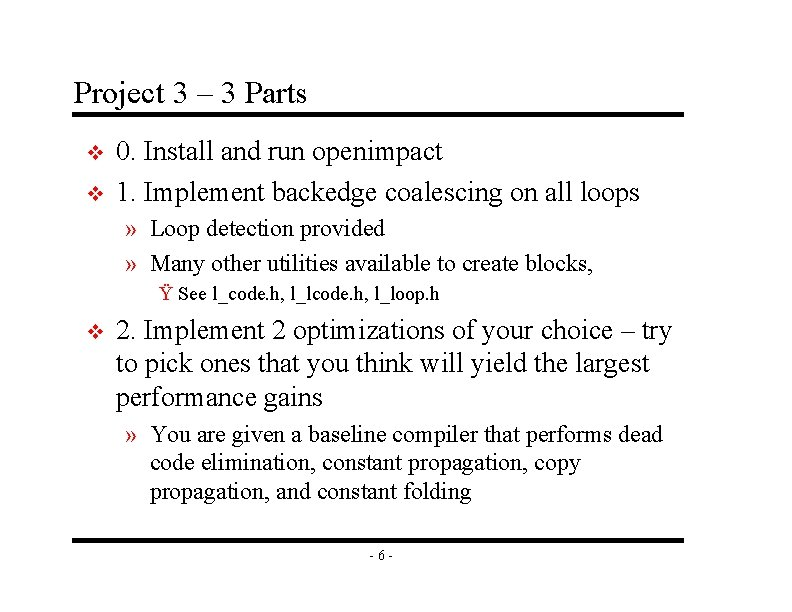Project 3 – 3 Parts v v 0. Install and run openimpact 1. Implement backedge coalescing on all loops » Loop detection provided » Many other utilities available to create blocks, Ÿ See l_code. h, l_loop. h v 2. Implement 2 optimizations of your choice – try to pick ones that you think will yield the largest performance gains » You are given a baseline compiler that performs dead code elimination, constant propagation, copy propagation, and constant folding -6 -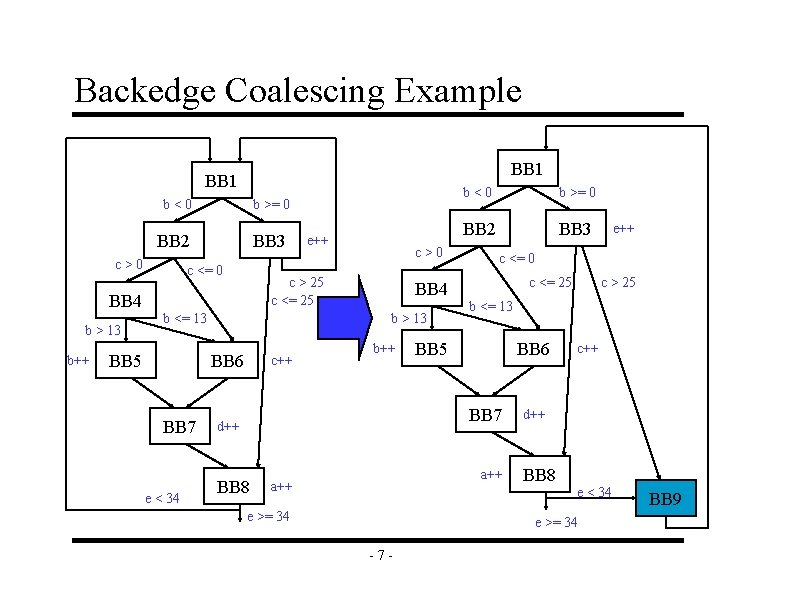Backedge Coalescing Example BB 1 b<0 b >= 0 BB 2 BB 3 c>0 BB 4 b > 13 b++ c <= 0 e < 34 BB 4 b > 13 BB 6 BB 7 c>0 c > 25 c <= 25 b <= 13 BB 5 e++ c++ b<0 b >= 0 BB 2 BB 3 c <= 0 c <= 25 BB 6 BB 8 a++ e >= 34 c > 25 b <= 13 BB 7 d++ c++ d++ BB 8 e < 34 e >= 34 -7 - e++ BB 9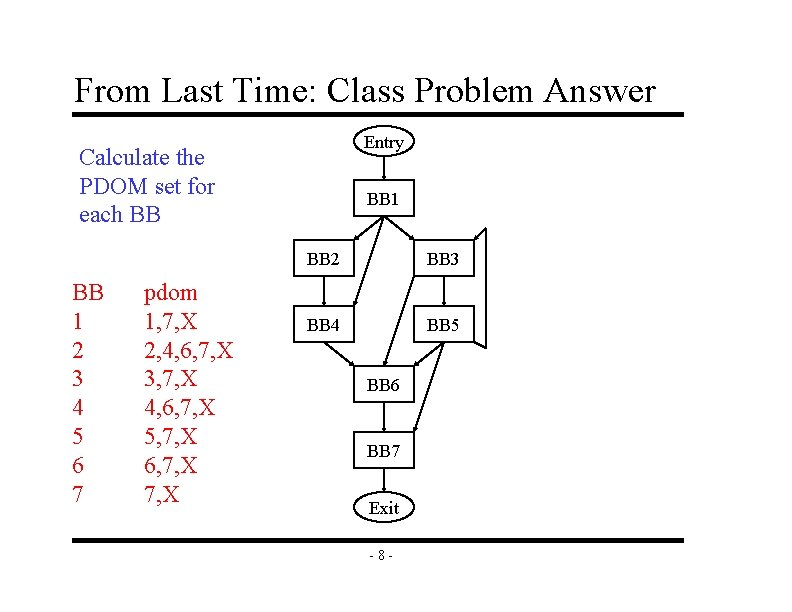From Last Time: Class Problem Answer Entry Calculate the PDOM set for each BB BB 1 2 3 4 5 6 7 pdom 1, 7, X 2, 4, 6, 7, X 3, 7, X 4, 6, 7, X 5, 7, X 6, 7, X BB 1 BB 2 BB 3 BB 4 BB 5 BB 6 BB 7 Exit -8 -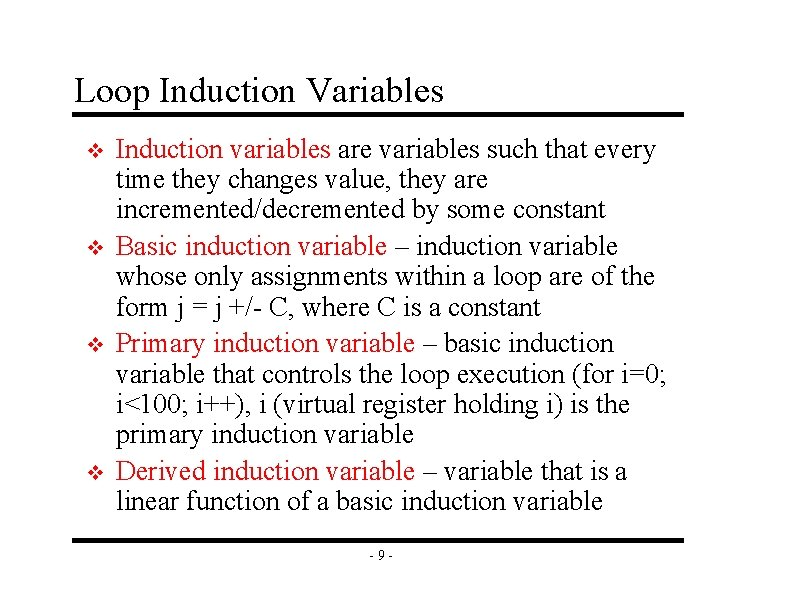Loop Induction Variables v v Induction variables are variables such that every time they changes value, they are incremented/decremented by some constant Basic induction variable – induction variable whose only assignments within a loop are of the form j = j +/- C, where C is a constant Primary induction variable – basic induction variable that controls the loop execution (for i=0; i<100; i++), i (virtual register holding i) is the primary induction variable Derived induction variable – variable that is a linear function of a basic induction variable -9 -Class Problem Identify the basic, primary, and derived inductions variables in this loop. r 1 = 0 r 7 = &A Loop: r 2 = r 1 * 4 r 4 = r 7 + 3 r 7 = r 7 + 1 r 1 = load(r 2) r 3 = load(r 4) r 9 = r 1 * r 3 r 10 = r 9 >> 4 store (r 10, r 2) r 1 = r 1 + 4 blt r 1 100 Loop - 10 -Reducible Flow Graphs v A flow graph is reducible if and only if we can partition the edges into 2 disjoint groups often called forward and back edges with the following properties » The forward edges form an acyclic graph in which every node can be reached from the Entry » The back edges consist only of edges whose destinations dominate their sources v More simply – Take a CFG, remove all the backedges (x y where y dominates x), you should have a connected, acyclic graph - 11 -Irreducible Flow Graph Example * In C/C++, its not possible to create an irreducible flow graph without using goto’s * Cyclic graphs that are NOT natural loops cannot be optimized by the compiler L 1: x = x + 1 if (x) { L 2: y = y + 1 if (y > 10) goto L 3 } else { L 3: z = z + 1 if (z > 0) goto L 2 } bb 1 bb 2 - 12 - Non-reducible! bb 3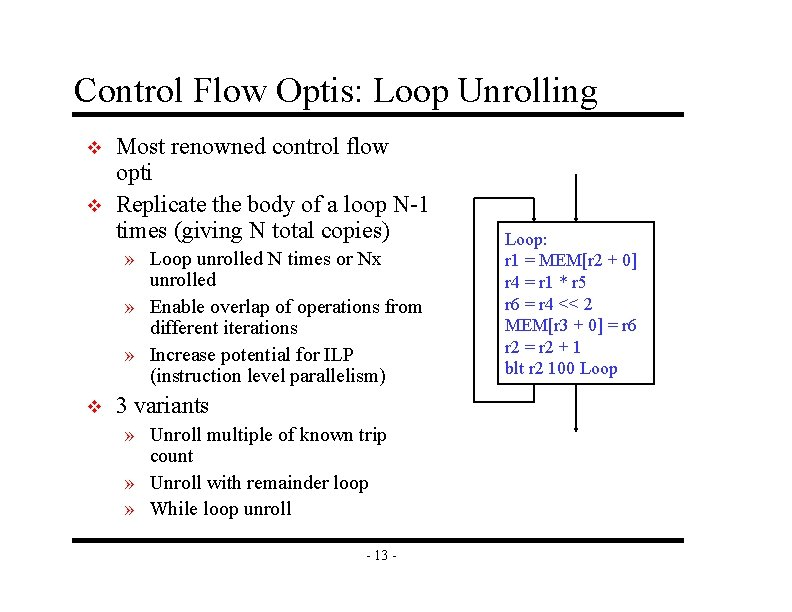Control Flow Optis: Loop Unrolling v v Most renowned control flow opti Replicate the body of a loop N-1 times (giving N total copies) » Loop unrolled N times or Nx unrolled » Enable overlap of operations from different iterations » Increase potential for ILP (instruction level parallelism) v 3 variants » Unroll multiple of known trip count » Unroll with remainder loop » While loop unroll - 13 - Loop: r 1 = MEM[r 2 + 0] r 4 = r 1 * r 5 r 6 = r 4 << 2 MEM[r 3 + 0] = r 6 r 2 = r 2 + 1 blt r 2 100 Loop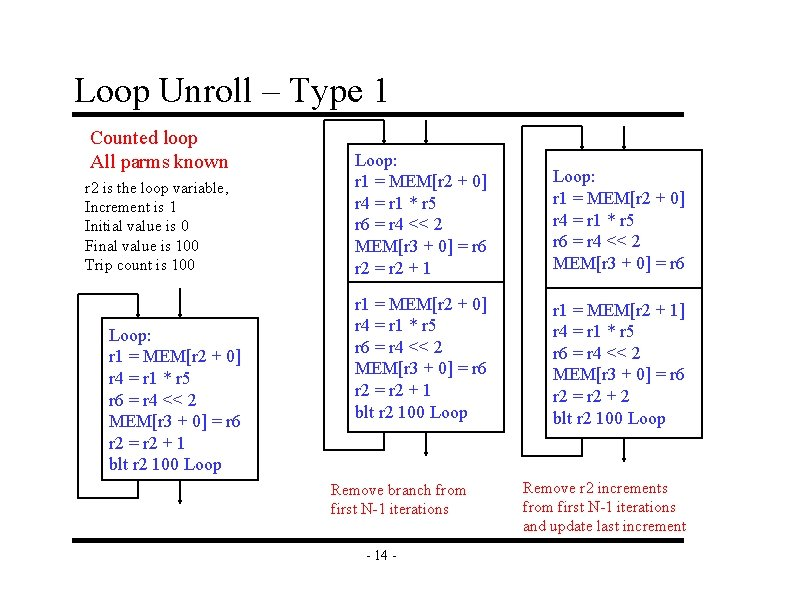Loop Unroll – Type 1 Counted loop All parms known r 2 is the loop variable, Increment is 1 Initial value is 0 Final value is 100 Trip count is 100 Loop: r 1 = MEM[r 2 + 0] r 4 = r 1 * r 5 r 6 = r 4 << 2 MEM[r 3 + 0] = r 6 r 2 = r 2 + 1 blt r 2 100 Loop: r 1 = MEM[r 2 + 0] r 4 = r 1 * r 5 r 6 = r 4 << 2 MEM[r 3 + 0] = r 6 r 2 = r 2 + 1 blt r 2 100 Loop r 1 = MEM[r 2 + 1] r 4 = r 1 * r 5 r 6 = r 4 << 2 MEM[r 3 + 0] = r 6 r 2 = r 2 + 2 blt r 2 100 Loop Remove branch from first N-1 iterations - 14 - Remove r 2 increments from first N-1 iterations and update last incrementLoop Unroll – Type 2 Counted loop Some parms unknown r 2 is the loop variable, Increment is ? Initial value is ? Final value is ? Trip count is ? Loop: r 1 = MEM[r 2 + 0] r 4 = r 1 * r 5 r 6 = r 4 << 2 MEM[r 3 + 0] = r 6 r 2 = r 2 + X blt r 2 Y Loop tc = final – initial tc = tc / increment rem = tc % N fin = rem * increment Rem. Loop: r 1 = MEM[r 2 + 0] r 4 = r 1 * r 5 r 6 = r 4 << 2 MEM[r 3 + 0] = r 6 r 2 = r 2 + X blt r 2 fin Rem. Loop Remainder loop executes the “leftover” iterations - 15 - Loop: r 1 = MEM[r 2 + 0] r 4 = r 1 * r 5 r 6 = r 4 << 2 MEM[r 3 + 0] = r 6 r 1 = MEM[r 2 + X] r 4 = r 1 * r 5 r 6 = r 4 << 2 MEM[r 3 + 0] = r 6 r 2 = r 2 + (N*X) blt r 2 Y Loop Unrolled loop same as Type 1, and is guaranteed to execute a multiple of N times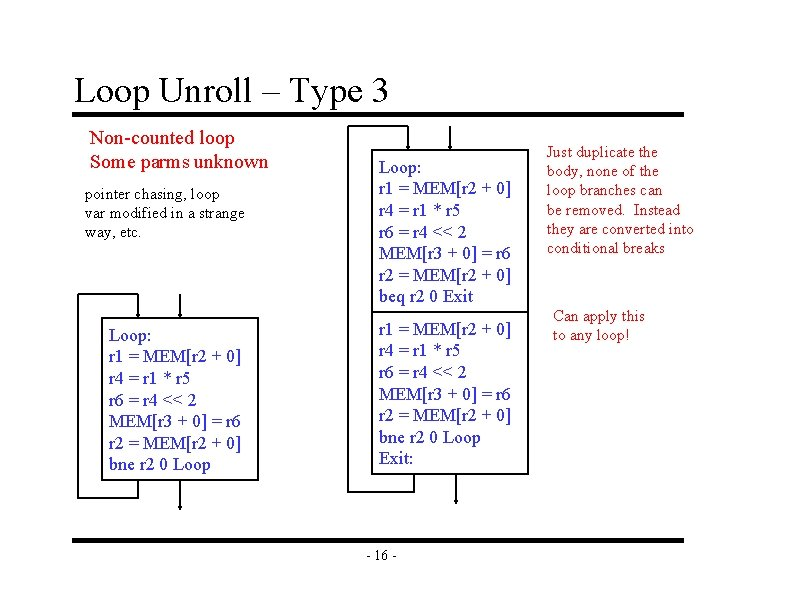Loop Unroll – Type 3 Non-counted loop Some parms unknown pointer chasing, loop var modified in a strange way, etc. Loop: r 1 = MEM[r 2 + 0] r 4 = r 1 * r 5 r 6 = r 4 << 2 MEM[r 3 + 0] = r 6 r 2 = MEM[r 2 + 0] bne r 2 0 Loop: r 1 = MEM[r 2 + 0] r 4 = r 1 * r 5 r 6 = r 4 << 2 MEM[r 3 + 0] = r 6 r 2 = MEM[r 2 + 0] beq r 2 0 Exit r 1 = MEM[r 2 + 0] r 4 = r 1 * r 5 r 6 = r 4 << 2 MEM[r 3 + 0] = r 6 r 2 = MEM[r 2 + 0] bne r 2 0 Loop Exit: - 16 - Just duplicate the body, none of the loop branches can be removed. Instead they are converted into conditional breaks Can apply this to any loop!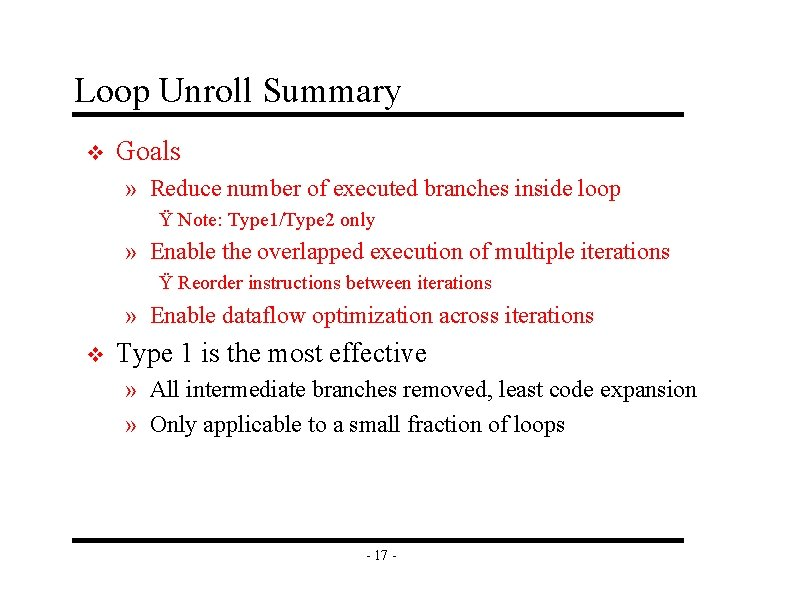Loop Unroll Summary v Goals » Reduce number of executed branches inside loop Ÿ Note: Type 1/Type 2 only » Enable the overlapped execution of multiple iterations Ÿ Reorder instructions between iterations » Enable dataflow optimization across iterations v Type 1 is the most effective » All intermediate branches removed, least code expansion » Only applicable to a small fraction of loops - 17 -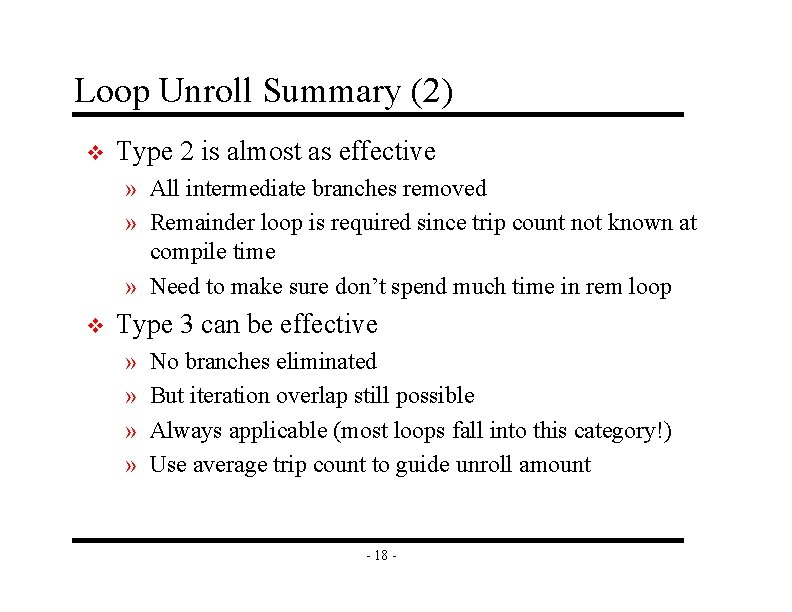Loop Unroll Summary (2) v Type 2 is almost as effective » All intermediate branches removed » Remainder loop is required since trip count not known at compile time » Need to make sure don’t spend much time in rem loop v Type 3 can be effective » » No branches eliminated But iteration overlap still possible Always applicable (most loops fall into this category!) Use average trip count to guide unroll amount - 18 -Class Problem Unroll both the outer loop and inner loop 2 x. Apply the most aggressive style unrolling that you can, e. g. , Type 1 if possible, else Type 2, else Type 3 for (i=0; i<100; i++) { j = i; while (j < 100) { A[j]--; } B[i] = 0; } - 19 -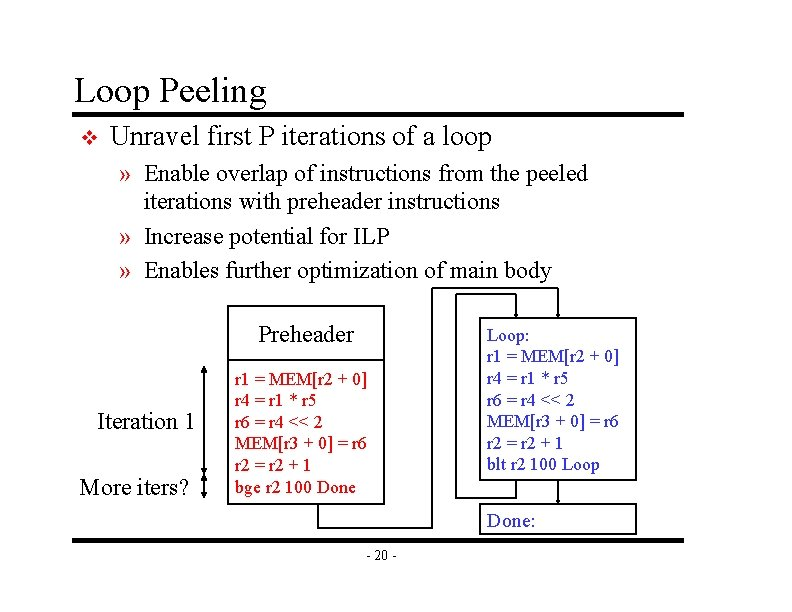Loop Peeling v Unravel first P iterations of a loop » Enable overlap of instructions from the peeled iterations with preheader instructions » Increase potential for ILP » Enables further optimization of main body Preheader Iteration 1 More iters? r 1 = MEM[r 2 + 0] r 4 = r 1 * r 5 r 6 = r 4 << 2 MEM[r 3 + 0] = r 6 r 2 = r 2 + 1 bge r 2 100 Done Loop: r 1 = MEM[r 2 + 0] r 4 = r 1 * r 5 r 6 = r 4 << 2 MEM[r 3 + 0] = r 6 r 2 = r 2 + 1 blt r 2 100 Loop Done: - 20 -Control Flow Opti for Acyclic Code v Rather simple transformations with these goals: » Reduce the number of dynamic branches » Make larger basic blocks » Reduce code size v Classic control flow optimizations » » » » Branch to unconditional branch Unconditional branch to branch Branch to next basic block Basic block merging Branch to same target Branch target expansion Unreachable code elimination - 21 -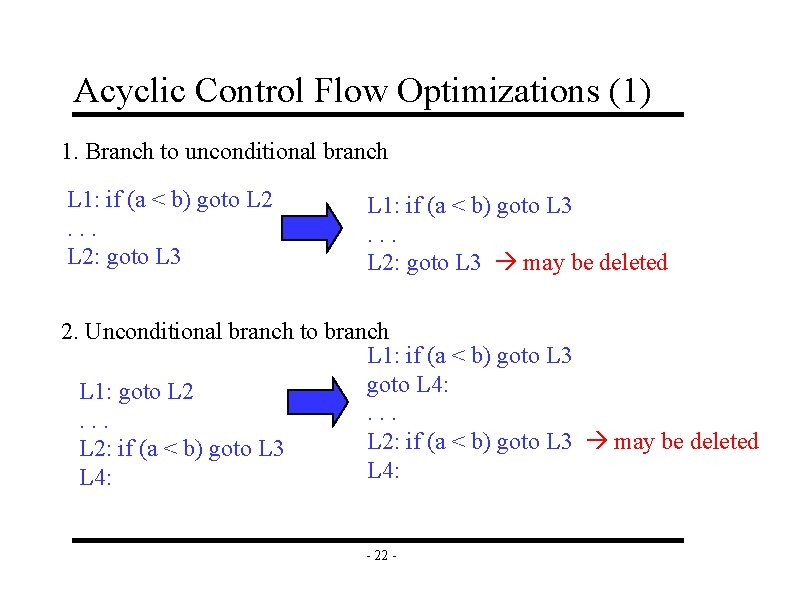Acyclic Control Flow Optimizations (1) 1. Branch to unconditional branch L 1: if (a < b) goto L 2. . . L 2: goto L 3 L 1: if (a < b) goto L 3. . . L 2: goto L 3 may be deleted 2. Unconditional branch to branch L 1: if (a < b) goto L 3 goto L 4: L 1: goto L 2. . . L 2: if (a < b) goto L 3 may be deleted L 2: if (a < b) goto L 3 L 4: - 22 -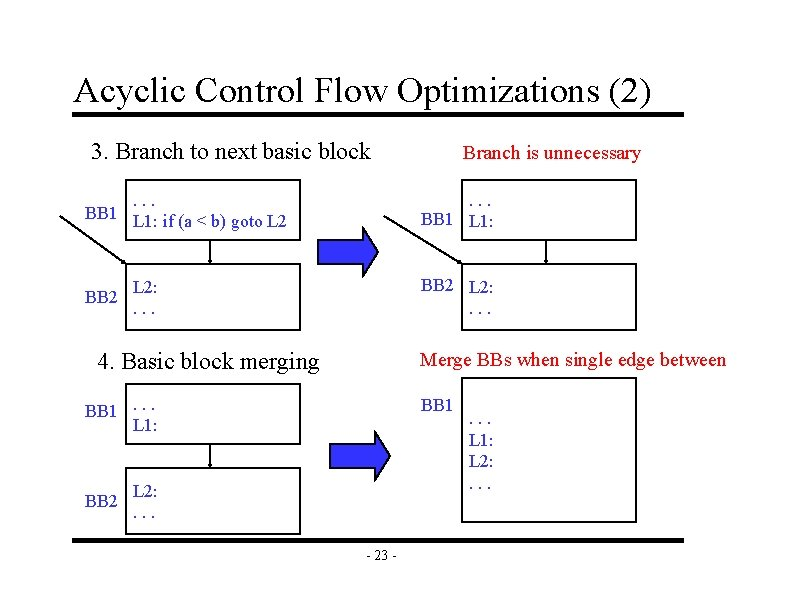Acyclic Control Flow Optimizations (2) 3. Branch to next basic block. . . BB 1 L 1: if (a < b) goto L 2 BB 2 . . . BB 1 L 1: BB 2 L 2: . . . 4. Basic block merging Merge BBs when single edge between BB 1. . . L 1: BB 2 Branch is unnecessary BB 1 L 2: . . . - 23 - . . . L 1: L 2: . . .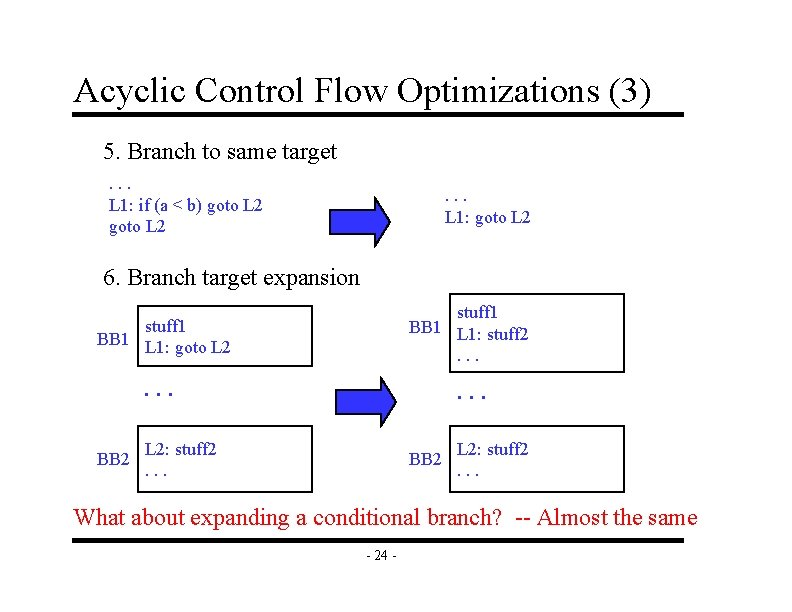Acyclic Control Flow Optimizations (3) 5. Branch to same target. . . L 1: if (a < b) goto L 2 . . . L 1: goto L 2 6. Branch target expansion stuff 1 BB 1 L 1: goto L 2 stuff 1 BB 1 L 1: stuff 2. . BB 2 L 2: stuff 2. . . What about expanding a conditional branch? -- Almost the same - 24 -Unreachable Code Elimination Algorithm entry Mark procedure entry BB visited to_visit = procedure entry BB while (to_visit not empty) { current = to_visit. pop() for (each successor block of current) { Mark successor as visited; to_visit += successor } } Eliminate all unvisited blocks - 25 - bb 1 bb 2 bb 3 bb 4 bb 5 Which BB(s) can be deleted?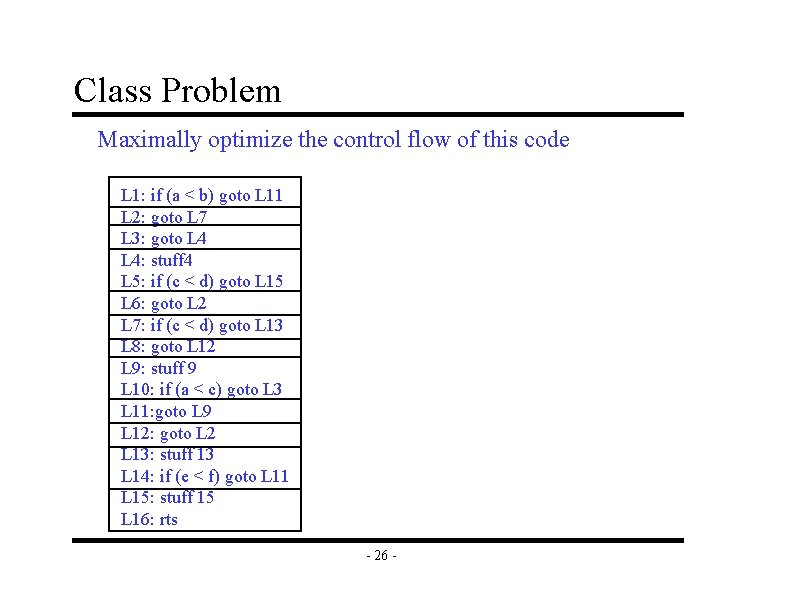Class Problem Maximally optimize the control flow of this code L 1: if (a < b) goto L 11 L 2: goto L 7 L 3: goto L 4: stuff 4 L 5: if (c < d) goto L 15 L 6: goto L 2 L 7: if (c < d) goto L 13 L 8: goto L 12 L 9: stuff 9 L 10: if (a < c) goto L 3 L 11: goto L 9 L 12: goto L 2 L 13: stuff 13 L 14: if (e < f) goto L 11 L 15: stuff 15 L 16: rts - 26 -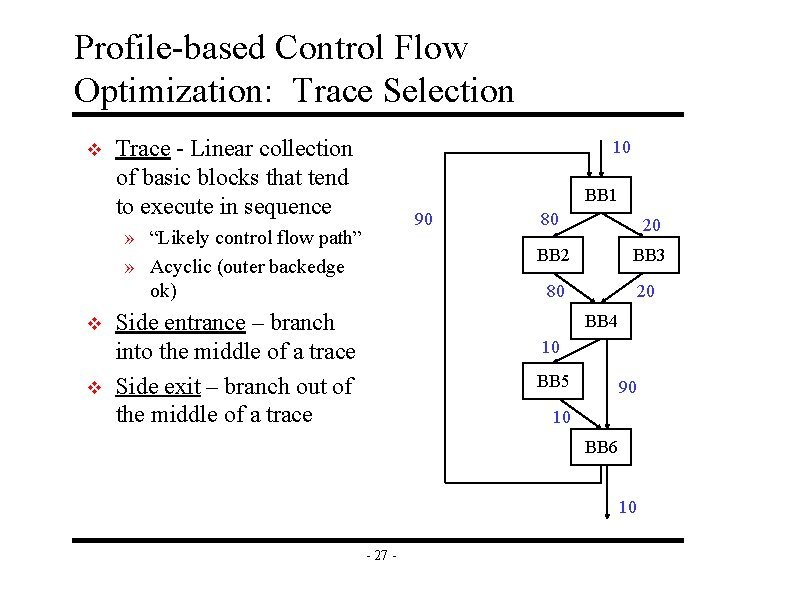Profile-based Control Flow Optimization: Trace Selection v Trace - Linear collection of basic blocks that tend to execute in sequence 10 BB 1 90 » “Likely control flow path” » Acyclic (outer backedge ok) v v Side entrance – branch into the middle of a trace Side exit – branch out of the middle of a trace 80 20 BB 2 BB 3 80 20 BB 4 10 BB 5 90 10 BB 6 10 - 27 -Linearizing a Trace 10 (entry count) BB 1 20 (side exit) 80 90 (entry/ exit count) BB 2 80 BB 3 20 (side entrance) BB 4 10 (side exit) BB 5 90 BB 6 10 (side entrance) 10 (exit count) - 28 -Intelligent Trace Layout for Icache Performance BB 1 BB 2 Intraprocedural code placement Procedure positioning Procedure splitting trace 1 trace 2 BB 4 BB 6 trace 3 BB 3 The rest BB 5 Procedure view Trace view - 29 -